# Introduction

The purpose of this vignette is to introduce the bdpbinomial function. bdpbinomial is used for estimating posterior samples from a Binomial event rate outcome for clinical trials where an informative prior is used. In the parlance of clinical trials, the informative prior is derived from historical data. The weight given to the historical data is determined using what we refer to as a discount function. There are three steps in carrying out estimation:

1. Estimation of the historical data weight, denoted $$\hat{\alpha}$$, via the discount function

2. Estimation of the posterior distribution of the current data, conditional on the historical data weighted by $$\hat{\alpha}$$

3. If a two-arm clinical trial, estimation of the posterior treatment effect, i.e., treatment versus control

Throughout this vignette, we use the terms current, historical, treatment, and control. These terms are used because the model was envisioned in the context of clinical trials where historical data may be present. Because of this terminology, there are 4 potential sources of data:

1. Current treatment data: treatment data from a current study

2. Current control data: control (or other treatment) data from a current study

3. Historical treatment data: treatment data from a previous study

4. Historical control data: control (or other treatment) data from a previous study

If only treatment data is input, the function considers the analysis a one-arm trial. If treatment data + control data is input, then it is considered a two-arm trial.

## Estimation of the historical data weight

In the first estimation step, the historical data weight $$\hat{\alpha}$$ is estimated. In the case of a two-arm trial, where both treatment and control data are available, an $$\hat{\alpha}$$ value is estimated separately for each of the treatment and control arms. Of course, historical treatment or historical control data must be present, otherwise $$\hat{\alpha}$$ is not estimated for the corresponding arm.

When historical data are available, estimation of $$\hat{\alpha}$$ is carried out as follows. Let $$y$$ and $$N$$ denote the number of events and sample size of the current data, respectively. Similarly, let $$y_0$$ and $$N_0$$ denote the number of events and sample size of the historical data, respectively. Let $$a_0$$ and $$b_0$$ denote the rate parameters of a Beta distribution. Then, the posterior distributions of the event rates for current and historical data, under vague (flat) priors are

$\tilde{\theta}\mid y,N \sim \mathcal{B}eta\left(y+a_0,\,N-y+b_0 \right)$ and

$\theta_0\mid y_0,N_0 \sim \mathcal{B}eta\left(y_0+a_0,\,N_0-y_0+b_0 \right),$ respectively. We next compute the posterior probability $$p = Pr\left(\tilde{\theta} \lt \theta_0\mid y, N, y_0, N_0\right)$$. Finally, for a discount function, denoted $$W$$, $$\hat{\alpha}$$ is computed as $\hat{\alpha} = \alpha_{max}\cdot W\left(p, \,w\right),\,0\le p\le1,$ where $$w$$ may be one or more parameters associated with the discount function and $$\alpha_{max}$$ scales the weight $$\hat{\alpha}$$ by a user-input maximum value. More details on the discount functions are given in the discount function section below.

There are several model inputs at this first stage. First, the user can select the discount function type via the discount_function input (see below). Next, choosing fix_alpha=TRUE forces a fixed value of $$\hat{\alpha}$$ (at the alpha_max input), as opposed to estimation via the discount function. In the next modeling stage, a Monte Carlo estimation approach is used, requiring several samples from the posterior distributions. Thus, the user can input a sample size greater than or less than the default value of number_mcmc=10000. Finally, the Beta rate parameters can be changed from the defaults of $$a_0=b_0=1$$ (a0 and b0 inputs).

An alternate Monte Carlo-based estimation scheme of $$\hat{\alpha}$$ has been implemented, controlled by the function input method="mc". Here, instead of treating $$\hat{\alpha}$$ as a fixed quantity, $$\hat{\alpha}$$ is treated as random. First, $$p$$, is computed as

$\begin{array}{rcl} v^2 & = & \displaystyle{\tilde{\theta}\left(1-\tilde{\theta}\right)/N} ,\\ \\ v^2_0 & = & \displaystyle{\theta_0\left(1-\theta_0\right)/N_0} ,\\ \\ Z & = & \displaystyle{\frac{\left|\tilde{\theta}-\theta_0\right|}{\sqrt{v^2 + v^2_0}}} ,\\ \\ p & = & 2\left(1-\Phi\left(Z\right)\right), \end{array}$ where $$\Phi\left(x\right)$$ is the $$x$$th quantile of a standard normal (i.e, the pnorm R function). Here, $$v^2$$ and $$v^2_0$$ are estimates of the variances of $$\tilde{\theta}$$ and $$\theta_0$$, respectively. Next, $$p$$ is used to construct $$\hat{\alpha}$$ via the discount function. Since the values $$Z$$ and $$p$$ are computed at each iteration of the Monte Carlo estimation scheme, $$\hat{\alpha}$$ is computed at each iteration of the Monte Carlo estimation scheme, resulting in a distribution of $$\hat{\alpha}$$ values.

### Discount function

There are currently three discount functions implemented throughout the bayesDP package. The discount function is specified using the discount_function input with the following choices available:

1. identity (default): Identity.

2. weibull: Weibull cumulative distribution function (CDF);

3. scaledweibull: Scaled Weibull CDF;

First, the identity discount function (default) sets the discount weight $$\hat{\alpha}=p$$.

Second, the Weibull CDF has two user-specified parameters associated with it, the shape and scale. The default shape is 3 and the default scale is 0.135, each of which are controlled by the function inputs weibull_shape and weibull_scale, respectively. The form of the Weibull CDF is $W(x) = 1 - \exp\left\{- (x/w_{scale})^{w_{shape}}\right\}.$

The third discount function option is the Scaled Weibull CDF. The Scaled Weibull CDF is the Weibull CDF divided by the value of the Weibull CDF evaluated at 1, i.e., $W^{\ast}(x) = W(x)/W(1).$ Similar to the Weibull CDF, the Scaled Weibull CDF has two user-specified parameters associated with it, the shape and scale, again controlled by the function inputs weibull_shape and weibull_scale, respectively.

Using the default shape and scale inputs, each of the discount functions are shown below.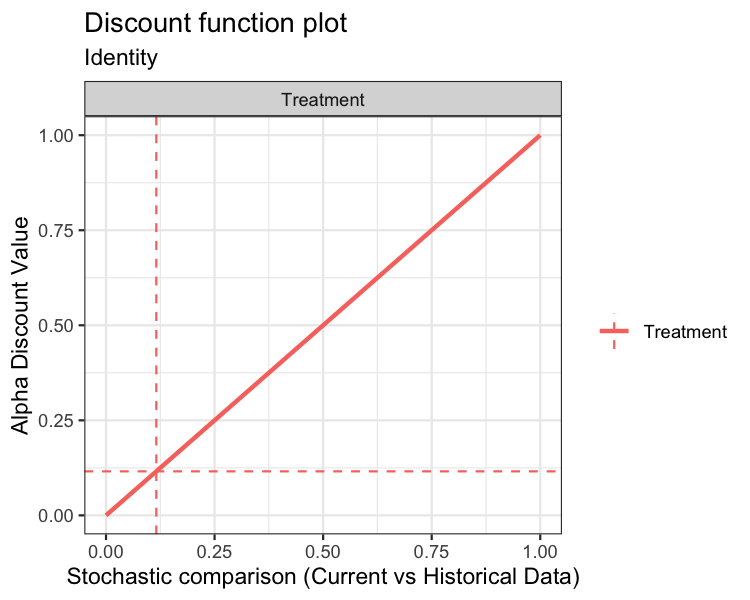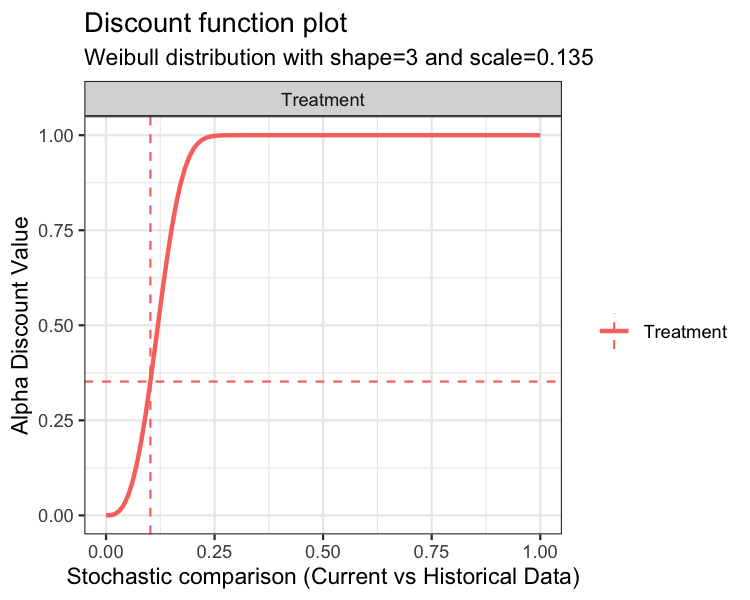In each of the above plots, the x-axis is the stochastic comparison between current and historical data, which we’ve denoted $$p$$. The y-axis is the discount value $$\hat{\alpha}$$ that corresponds to a given value of $$p$$.

An advanced input for the plot function is print. The default value is print = TRUE, which simply returns the graphics. Alternately, users can specify print = FALSE, which returns a ggplot2 object. Below is an example using the discount function plot:

p1 <- plot(fit02, type="discount", print=FALSE)
p1 + ggtitle("Discount Function Plot :-)")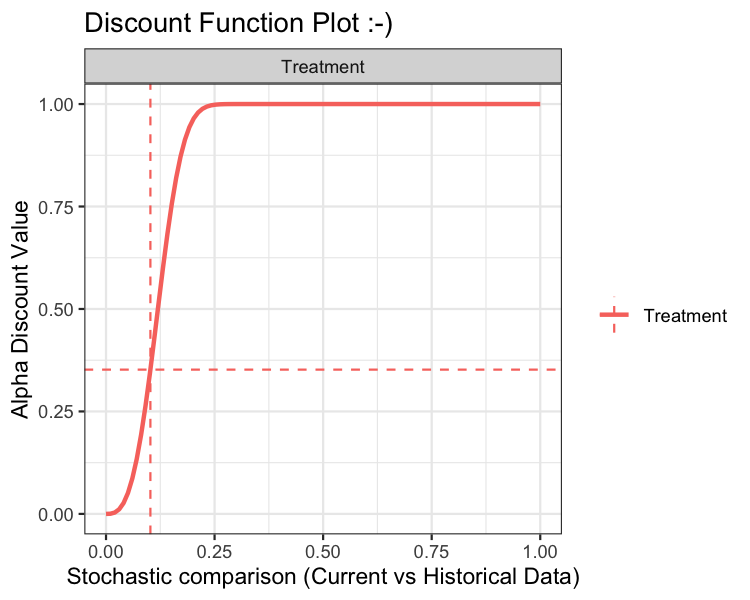## Estimation of the posterior distribution of the current data, conditional on the historical data

With $$\hat{\alpha}$$ in hand, we can now estimate the posterior distribution of the current data event rate. Using the notation of the previous section, the posterior distribution is $\theta \sim \mathcal{B}eta\left(y+y_0\hat{\alpha}+a_0,\, N-y+\hat{\alpha}(N_0-y_0)+b_0 \right).$ At this model stage, we have in hand number_mcmc simulations from the augmented event rate distribution. If there are no control data, i.e., a one-arm trial, then the modeling stops and we generate summaries of the posterior distribution of $$\theta$$. Otherwise, if there are control data, we proceed to a third step and compute a comparison between treatment and control data.

## Estimation of the posterior treatment effect: treatment versus control

This step of the model is carried out on-the-fly using the summary or print methods. Let $$\theta_T$$ and $$\theta_C$$ denote posterior event rate estimates of the treatment and control arms, respectively. Currently, the implemented comparison between treatment and control is the difference, i.e., summary statistics related to the posterior difference: $$\theta_T - \theta_C$$. In a future release, we may consider implementing additional comparison types.

## Inputting Data

The data inputs for bdpbinomial are y_t, N_t, y0_t, N0_t, y_c, N_c, y0_c, and N0_c. The data must be input as (y, N) pairs. For example, y_t, the number of events in the current treatment group, must be accompanied by N_t, the sample size of the current treatment group. Historical data inputs are not necessary, but using this function would not be necessary either.

At the minimum, y_t and N_t must be input. In the case that only y_t and N_t are input, the analysis is analogous to using prop.test. Each of the following input combinations are allowed:

• (y_t, N_t) - one-arm trial
• (y_t, N_t) + (y0_t, N0_t) - one-arm trial
• (y_t, N_t) + (y_c, N_c) - two-arm trial
• (y_t, N_t) + (y0_c, N0_c) - two-arm trial
• (y_t, N_t) + (y0_t, N0_t) + (y_c, N_c) - two-arm trial
• (y_t, N_t) + (y0_t, N0_t) + (y0_c, N0_c) - two-arm trial
• (y_t, N_t) + (y0_t, N0_t) + (y_c, N_c) + (y0_c, N0_c) - two-arm trial

# Examples

## One-arm trial

Suppose we have historical data with y0_t=25 events out of a sample size of N0_t=250 patients. This gives a historical event rate of 0.1. Now, suppose we have current data with y_t=15 events out of a sample size of N_t=200 patients, giving an event rate of 0.075. To illustrate the approach, let’s first give full weight to the historical data. This is accomplished by setting alpha_max=1 and fix_alpha=TRUE as follows:

set.seed(42)
fit1 <- bdpbinomial(y_t       = 15,
N_t       = 200,
y0_t      = 25,
N0_t      = 250,
alpha_max = 1,
fix_alpha = TRUE,
method    = "fixed")
summary(fit1)
##
##     One-armed bdp binomial
##
## Current treatment data: 15 and 200
## Historical treatment data: 25 and 250
## Stochastic comparison (p_hat) - treatment (current vs. historical data): 0.3664
## Discount function value (alpha) - treatment: 1
## 95 percent CI:
##  0.0663  0.1194
## sample estimates:
##  0.0902

Based on the summary output of fit1, we can see that the value of alpha was held fixed at 1. The resulting (augmented) event rate was estimated at 0.0902 which is approximately the event rate if we combined the historical and current data together, i.e., (15 + 25) / (200 + 250) = 0.089. Note that the print and summary methods result in the same output.

Now, let’s relax the constraint on fixing alpha at 1. We’ll also take this opportunity to describe the output of the plot method.

set.seed(42)
fit1a <- bdpbinomial(y_t       = 15,
N_t       = 200,
y0_t      = 25,
N0_t      = 250,
alpha_max = 1,
fix_alpha = FALSE,
method    = "fixed")
summary(fit1a)
##
##     One-armed bdp binomial
##
## Current treatment data: 15 and 200
## Historical treatment data: 25 and 250
## Stochastic comparison (p_hat) - treatment (current vs. historical data): 0.3664
## Discount function value (alpha) - treatment: 0.3664
## 95 percent CI:
##  0.0566  0.1211
## sample estimates:
##  0.085

When alpha is not constrained to one, it is estimated based on a comparison between the current and historical data. We see that the stochastic comparison, p_hat, between historical and control is 0.3664. Here, p_hat is the posterior probability of the comparison between current and historical data. With the present example, p_hat = 0.3664 implies that the current and historical event rates are moderately different. The result is that the weight given to the historical data is shrunk towards zero. Thus, the estimate of alpha from the discount function is 0.3664, and the augmented posterior estimate of the event rate moves up from 0.075 observed in the current data to a value closer to 0.089.

Many of the the values presented in the summary method are accessible from the fit object. For instance, alpha is found in fit1a$posterior_treatment$alpha_discount and p_hat is located at fit1a$posterior_treatment$p_hat. The sample estimates and CI are computed at run-time. The results can be replicated as:

mean_augmented <- round(median(fit1a$posterior_treatment$posterior),4)
mean_augmented
##  0.085
CI95_augmented <- round(quantile(fit1a$posterior_treatment$posterior, prob=c(0.025, 0.975)),4)
CI95_augmented
##   2.5%  97.5%
## 0.0566 0.1211

Finally, we’ll explore the plot method.

plot(fit1a, type="posteriors")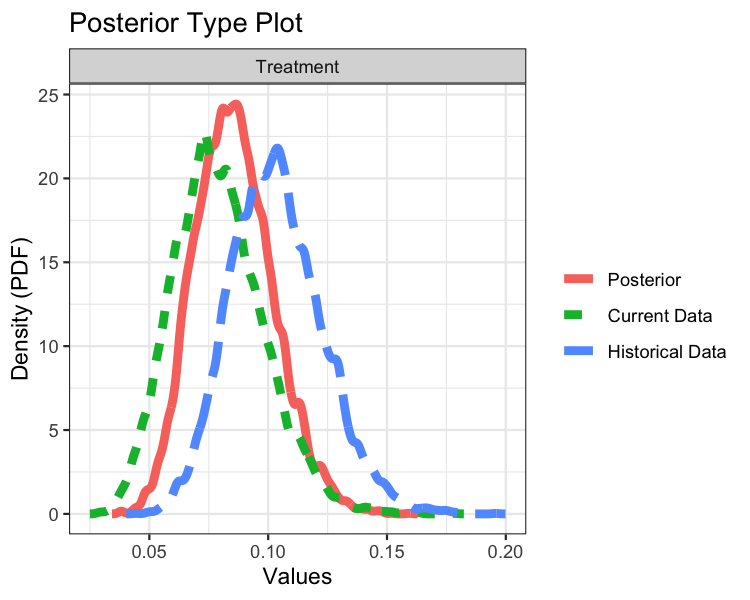plot(fit1a, type="density")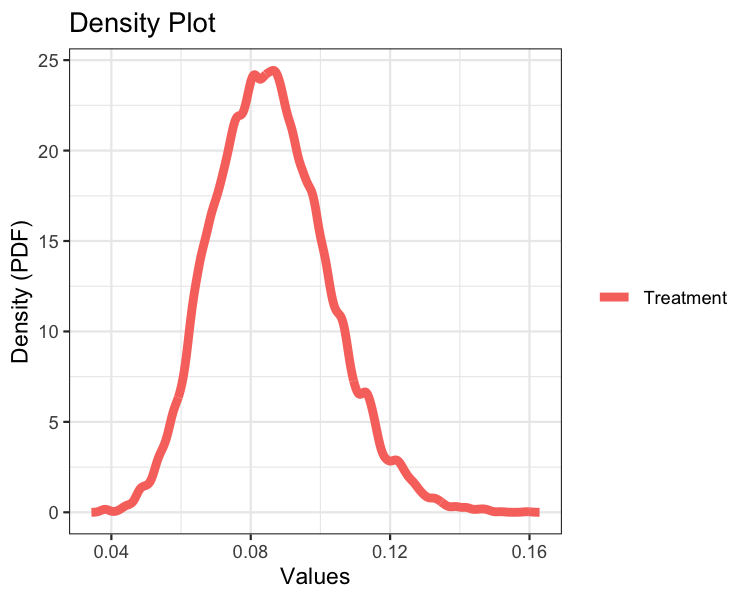plot(fit1a, type="discount")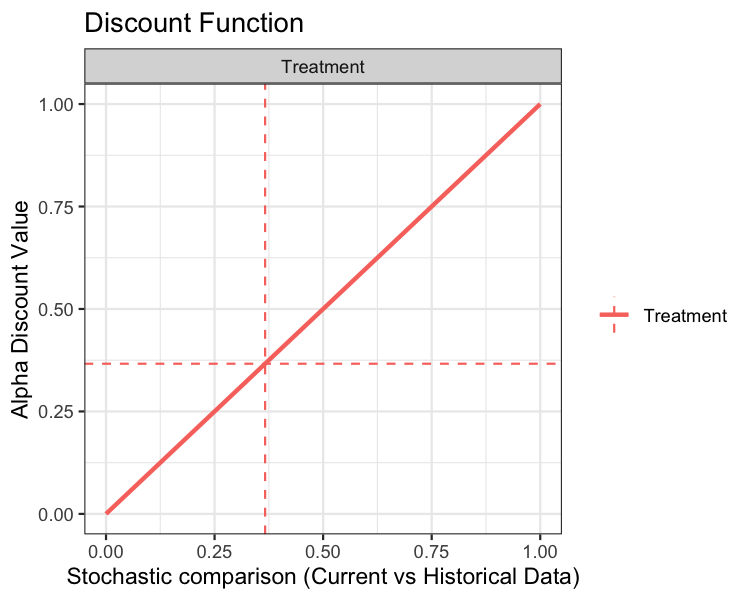The top plot displays three density curves. The blue curve is the density of the historical event rate, the green curve is the density of the current event rate, and the red curve is the density of the current event rate augmented by historical data. Since little weight was given to the historical data, the current and posterior event rates essentially overlap.

The middle plot simply re-displays the posterior event rate.

The bottom plot displays the discount function (solid curve) as well as alpha (horizontal dashed line) and p_hat (vertical dashed line). In the present example, the discount function is the identity.

## Two-arm trial

On to two-arm trials. In this package, we define a two-arm trial as an analysis where a current and/or historical control arm is present. Suppose we have the same treatment data as in the one-arm example, but now we introduce control data: y_c = 15, N_c = 200, y0_c = 20, and N0_c = 250. This control data gives a current and historical event rate of 20/250 = 0.08.

Before proceeding, it is worth pointing out that the discount function is applied separately to the treatment and control data. Now, let’s carry out the two-arm analysis using default inputs:

set.seed(42)
fit2 <- bdpbinomial(y_t  = 15,
N_t  = 200,
y0_t = 25,
N0_t = 250,
y_c  = 20,
N_c  = 250,
y0_c = 20,
N0_c = 250,
method = "fixed")
summary(fit2)
##
##     Two-armed bdp binomial
##
## Current treatment data: 15 and 200
## Current control data: 20 and 250
## Historical treatment data: 25 and 250
## Historical control data: 20 and 250
## Stochastic comparison (p_hat) - treatment (current vs. historical data): 0.3664
## Discount function value (alpha) - treatment: 0.3664
## Stochastic comparison (p_hat) - control (current vs. historical data): 0.9914
## Discount function value (alpha) - control: 0.9914
## alternative hypothesis: two.sided
## 95 percent CI:
##   -0.0347 0.0453
## sample estimates:
## prop 1 prop2
##   0.08  0.08

The summary method of a two-arm analysis is slightly different than a one-arm analysis. First, we see p_hat and alpha reported for the control data. In the present analysis, the current and historical control data have event rates that are very close, thus the historical control data is given full weight. This implies that the (augmented) posterior control event rate is approximately (20 + 20)/(250 + 250) = 0.08. Again, moderate weight is given to the historical treatment data, so we have an (augmented) posterior treatment event rate of 0.08.

The CI is computed at run time and is the interval estimate of the difference between the posterior treatment and control event rates. With a 95% CI of (-0.0347, 0.0453), we would conclude that the treatment and control arms are not significantly different.

The plot method of a two-arm analysis is slightly different than a one-arm analysis as well:

plot(fit2, type="posteriors")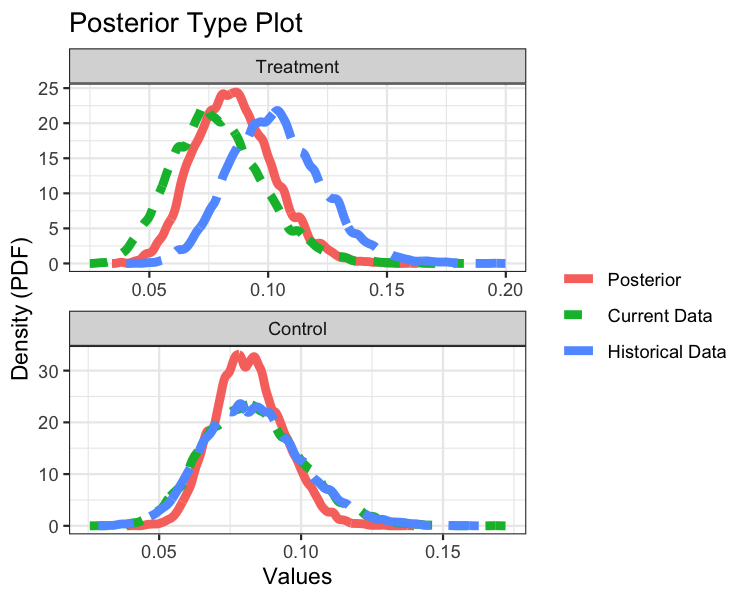plot(fit2, type="density")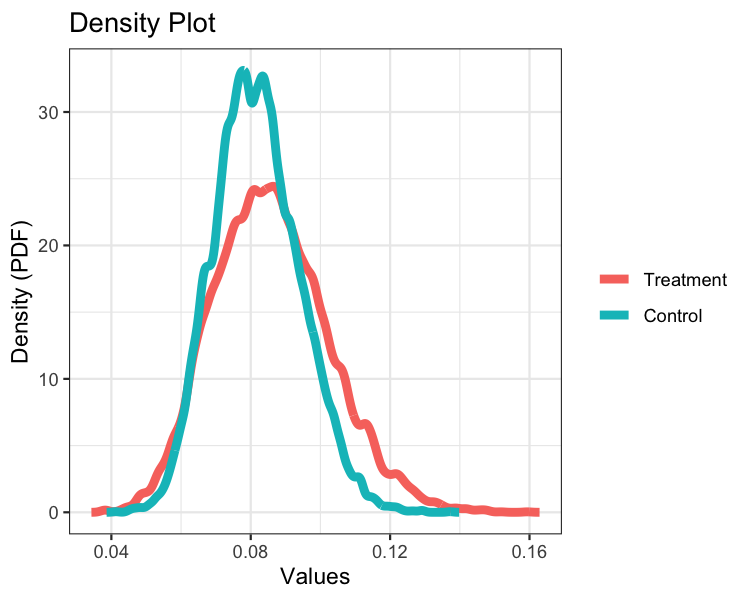plot(fit2, type="discount")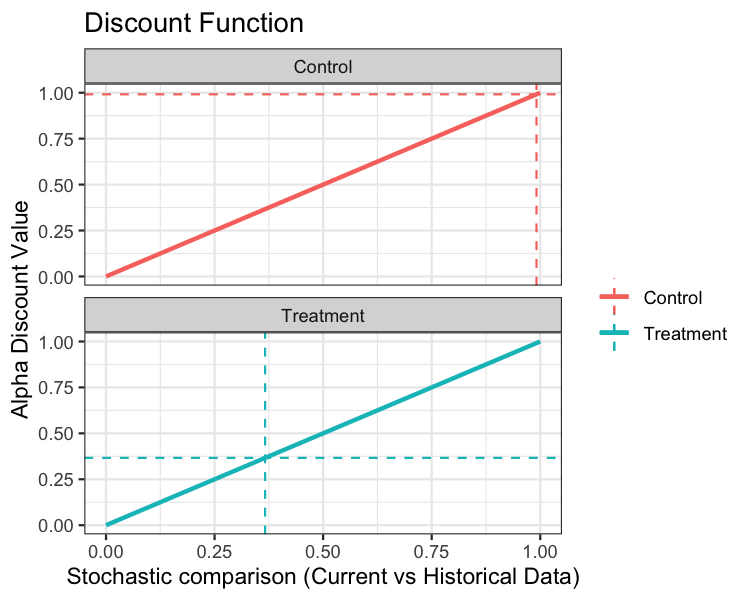Each of the three plots are analogous to the one-arm analysis, but each plot now presents additional data related to the control arm.